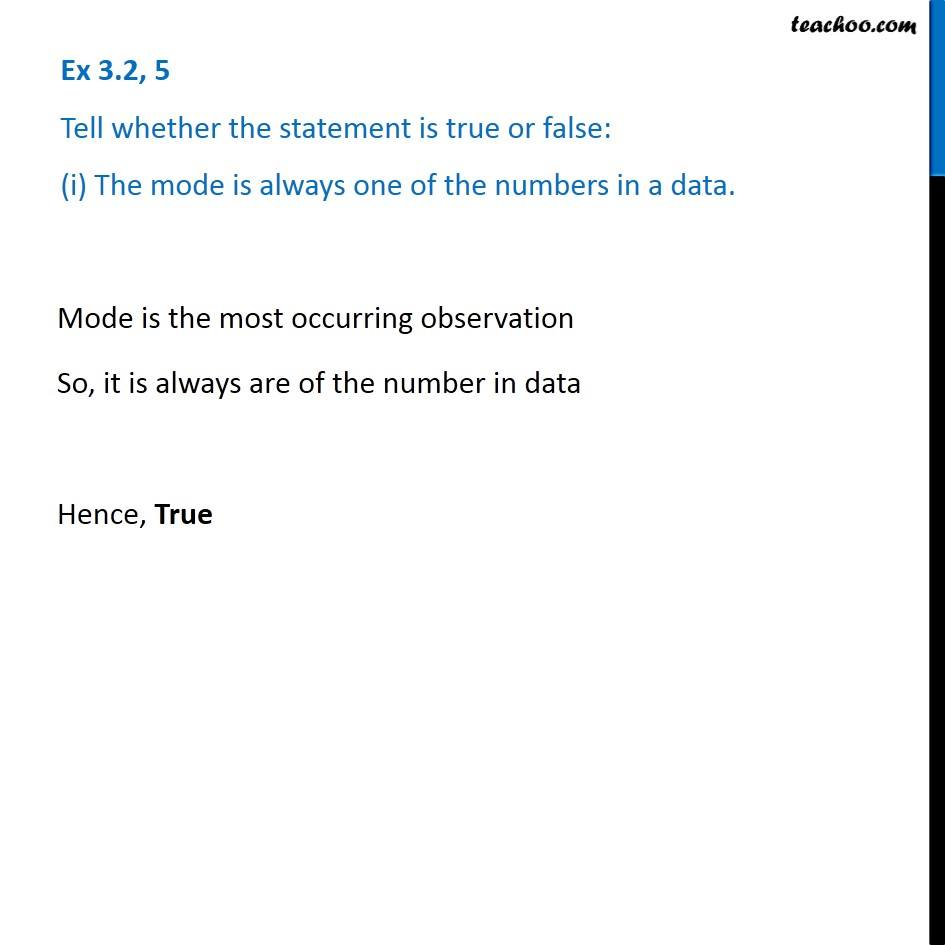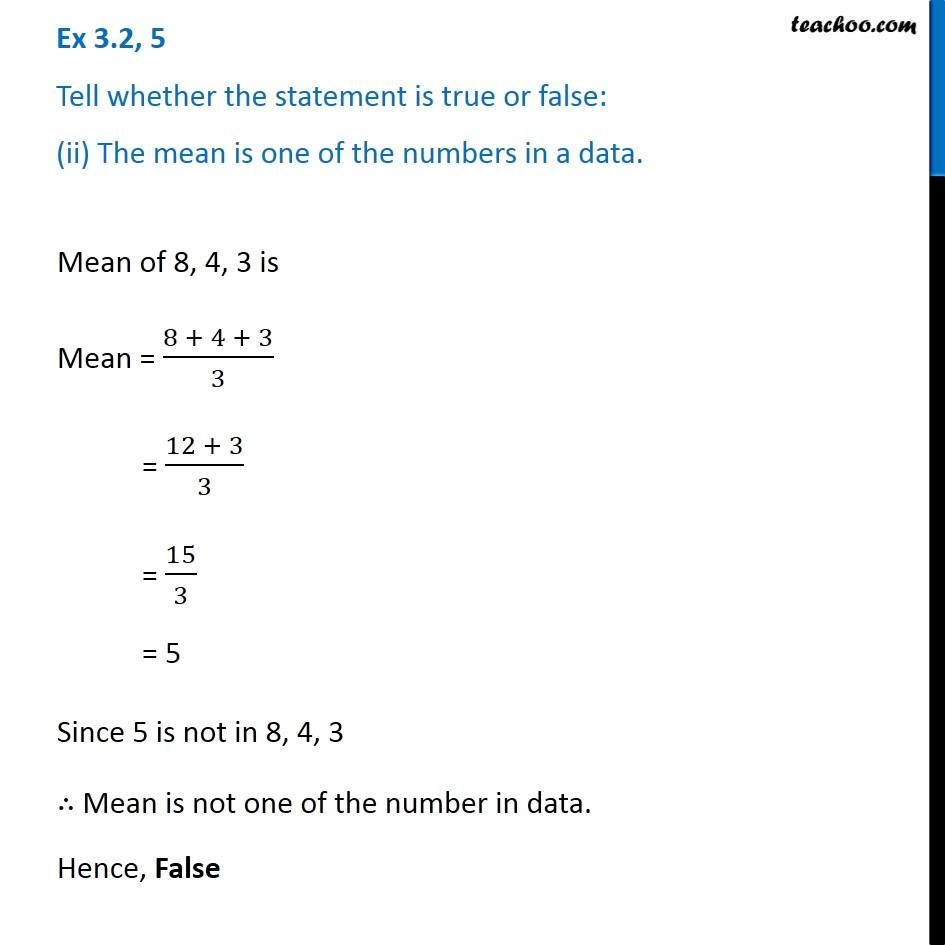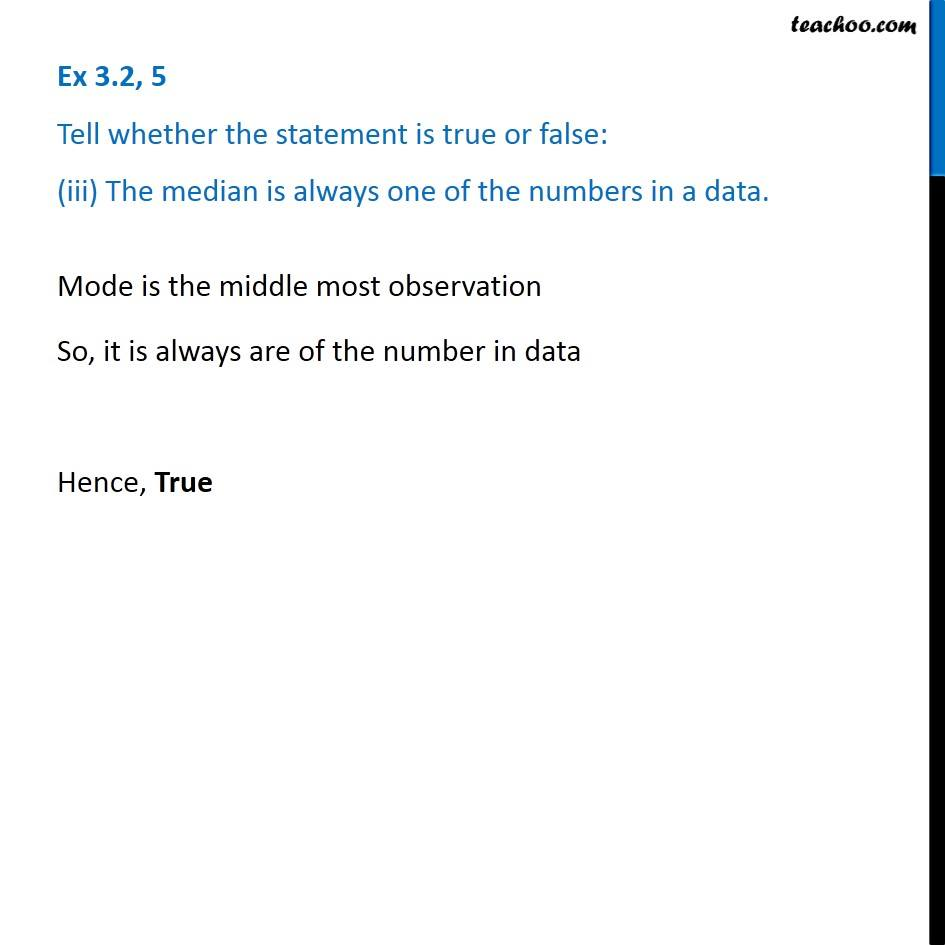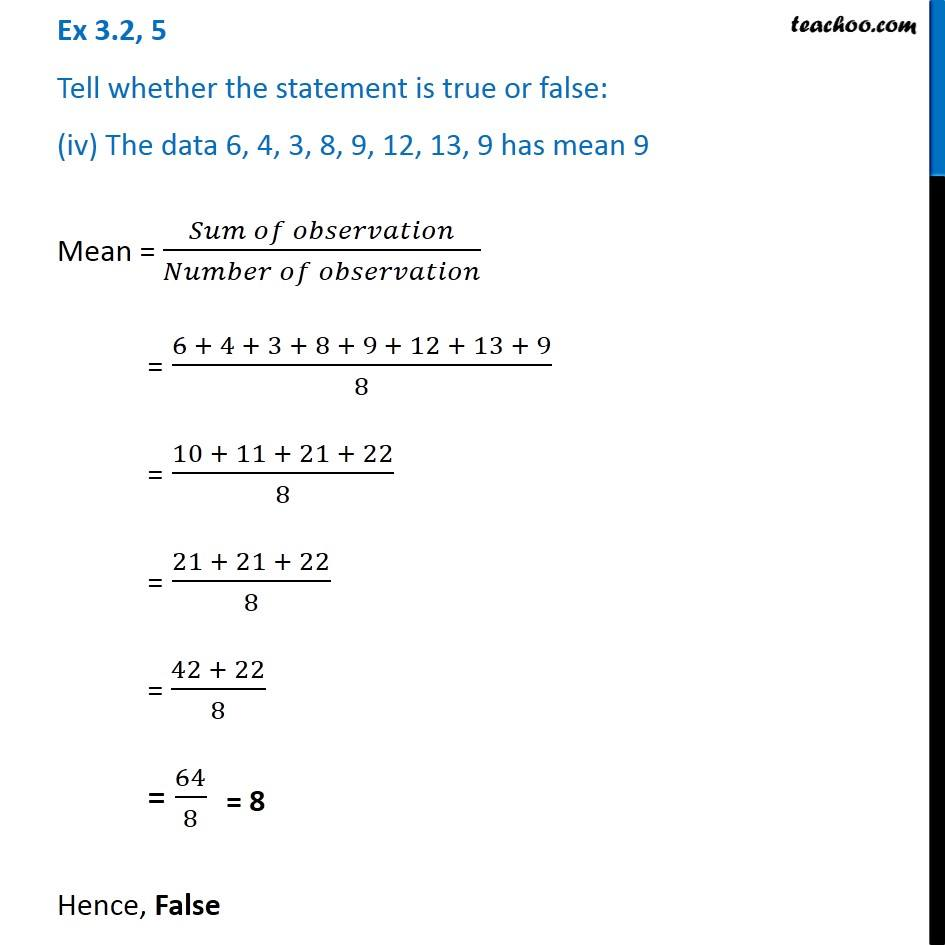Subscribe to our Youtube Channel - https://you.tube/teachoo

1. Chapter 3 Class 7 Data Handling
2. Serial order wise
3. Ex 3.2

Transcript

Ex 3.2, 5 Tell whether the statement is true or false: (i) The mode is always one of the numbers in a data. Mode is the most occurring observation So, it is always are of the number in data Hence, True Ex 3.2, 5 Tell whether the statement is true or false: (ii) The mean is one of the numbers in a data. Mean of 8, 4, 3 is Mean = (8 + 4 + 3)/3 = (12 + 3)/3 = 15/3 = 5 Since 5 is not in 8, 4, 3 ∴ Mean is not one of the number in data. Hence, False Ex 3.2, 5 Tell whether the statement is true or false: (iii) The median is always one of the numbers in a data. Mode is the middle most observation So, it is always are of the number in data Hence, True Ex 3.2, 5 Tell whether the statement is true or false: (iv) The data 6, 4, 3, 8, 9, 12, 13, 9 has mean 9 Mean = (𝑆𝑢𝑚 𝑜𝑓 𝑜𝑏𝑠𝑒𝑟𝑣𝑎𝑡𝑖𝑜𝑛)/(𝑁𝑢𝑚𝑏𝑒𝑟 𝑜𝑓 𝑜𝑏𝑠𝑒𝑟𝑣𝑎𝑡𝑖𝑜𝑛) = (6 + 4 + 3 + 8 + 9 + 12 + 13 + 9)/8 = (10 + 11 + 21 + 22)/8 = (21 + 21 + 22)/8 = (42 + 22)/8 = 64/8 Hence, False

Ex 3.2